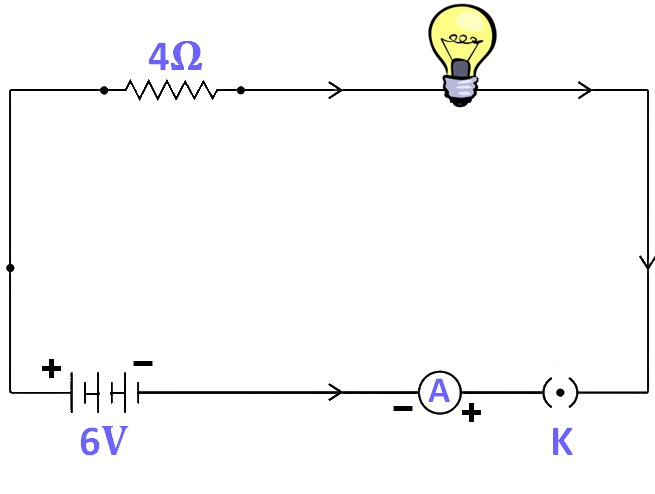Past Year - 5 Mark Questions

Class 10
Chapter 12 Class 10 - Electricity

## (d) power of the lamp.Given,

• Resistance of electric lamp, R 1 = 20 ohm
• Resistance of conductor, R 2 = 4 ohm
• Potential difference, V = 6 V

(a)

Since R 1 and R 2 are connected in series,

Total resistance, R = R 1 + R 2

= 20 + 4

= 24 ohm

Therefore, total resistance of the circuit is 24 ohm.

(b)

Let the current through the circuit be I.

According to ohm’s law,

V = I*R

I = V / R

= 6 / 24

= 0.25 A

Therefore, the current through the circuit is 0.25 A

(c)

(i) For electric lamp,

V = I*R 1

= 0.25 * 20

= 5 V

Therefore, the potential difference across the electric lamp is 5 V.

(ii) For conductor,

V = I*R 2

= 0.25 * 4

= 1 V

Therefore, the potential difference across the conductor is 1 V.

(d)

Let the power of the lamp be P.

We know that,

P = V*I

= 5 * 0.25

= 1.25 W

Therefore, power of the lamp is 1 . 25 W

Learn in your speed, with individual attention - Teachoo Maths 1-on-1 Class